# The uniform solid block in right figure has mass 0.2 kg and edge lengths a =...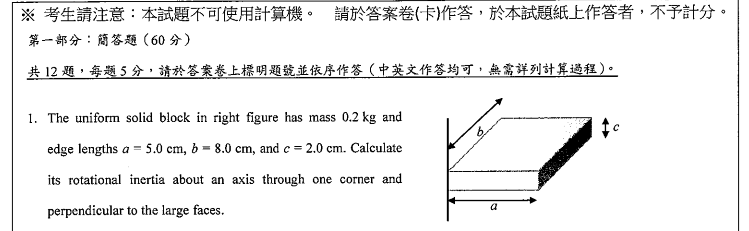The uniform solid block in right figure has mass 0.2 kg and edge lengths a = 5.0 cm, b = 8.0 cm, and c = 2.0 cm. Calculate its rotational inertia about an axis through one corner and perpendicular to the large faces.

The moment of inertia at the midddle of center= 1/12 M( b^2 + a^2)= 0.2/ 12 ( 0.0025 + 0.0064) = 1.483 x 10^-4

Distance between the center and axis at corner = 1/2 sqroot ( a^2+b^2)

Using the paralllel axis theorem,

I = 1/12 M ( b^2 +a^2) + M ( a^2+b^2) / 4 = 1.483 x 10^-4 + 4.45 x 10^-4= 5.933 x 10^-4

#### Earn Coin

Coins can be redeemed for fabulous gifts.

Similar Homework Help Questions
• ### The figure shows a uniform solid block of mass M = 9.4 kg and edge lengths...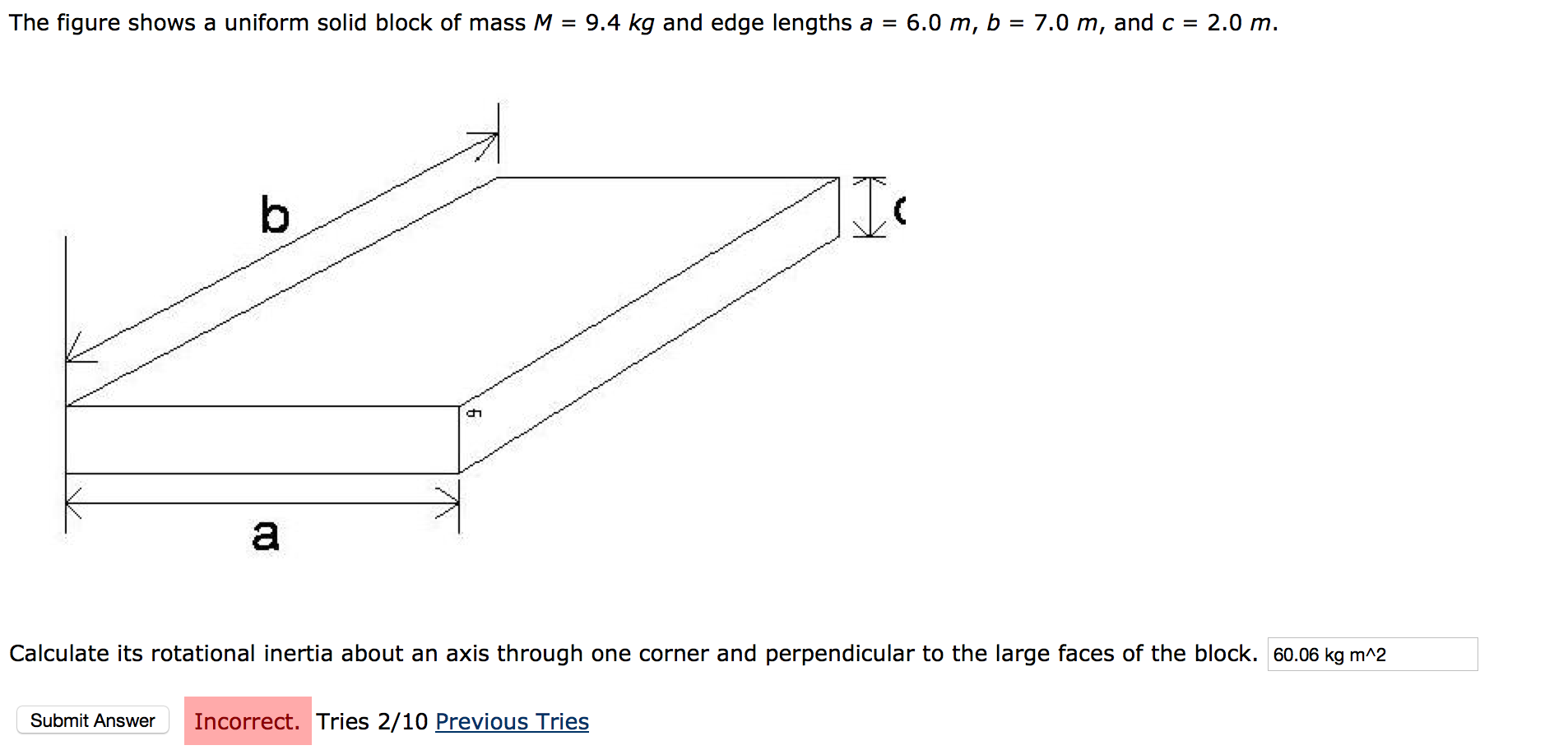The figure shows a uniform solid block of mass M = 9.4 kg and edge lengths a = 6.0 m, b = 7.0 m, and c = 2.0 m. Calculate its rotational inertia about an axis through one corner and perpendicular to the large faces of the block.

• ### The uniform solid block in the figure has mass 33.1 kg and edge lengths a =...The uniform solid block in the figure has mass 33.1 kg and edge lengths a = 0.853 m, b = 1.77 m, and c = 0.130 m. Calculate its rotational inertia about an axis through one corner and perpendicular to the large faces.

• ### The uniform solid block in the figure has mass 45.5 kg and edge lengths a =...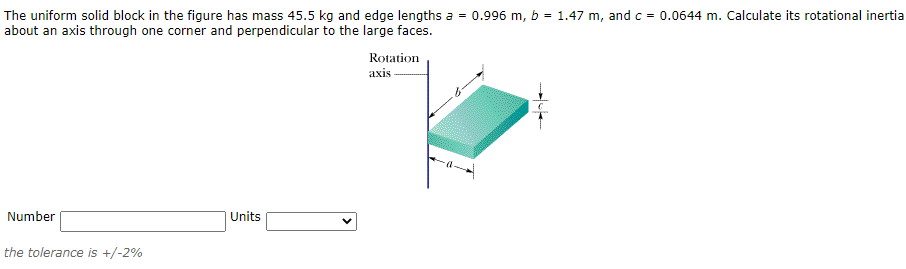The uniform solid block in the figure has mass 45.5 kg and edge lengths a = 0.996 m, b = 1.47 m, and c = 0.0644 m. Calculate its rotational inertia about an axis through one corner and perpendicular to the large faces. Rotation axis Number Units the tolerance is +/-2%

• ### The uniform solid block in the figure has mass 21.8 kg and edge lengths a 0.785m,...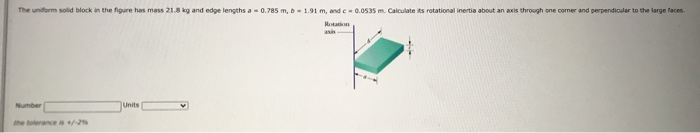The uniform solid block in the figure has mass 21.8 kg and edge lengths a 0.785m, b -1.91 m, and c-0.0535 m. Calculate its rotational inertia about an axis through one comer and perpendicular to the large faces. M Number Units the once

• ### The uniform solid block in Fig. 11-36 has a mass of M = 5.35 kg and...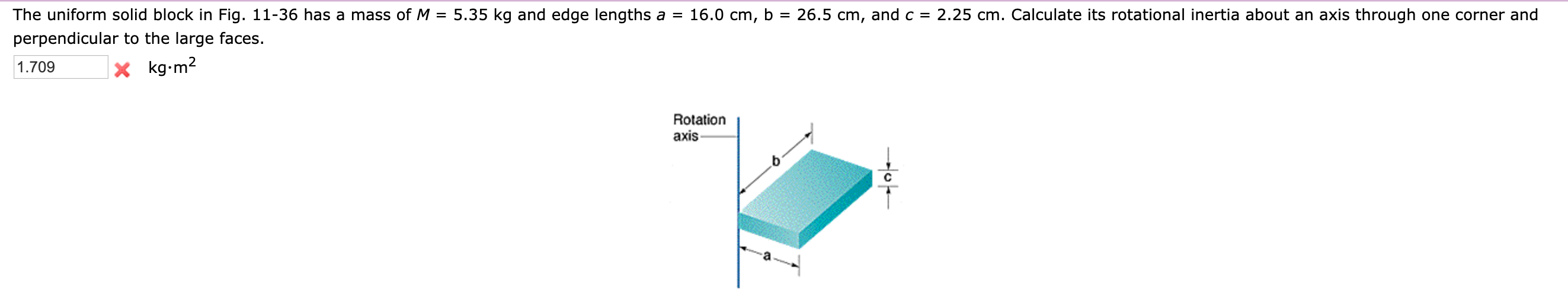The uniform solid block in Fig. 11-36 has a mass of M = 5.35 kg and edge lengths a = 16.0 cm, b = 26.5 cm, and c = 2.25 cm. Calculate its rotational inertia about an axis through one corner and perpendicular to the large faces. 1.709 x kg•m2 Rotation axis

• ### In your physics lab you are given a 11.7-kg uniform rectangular plate with edge lengths 64.7...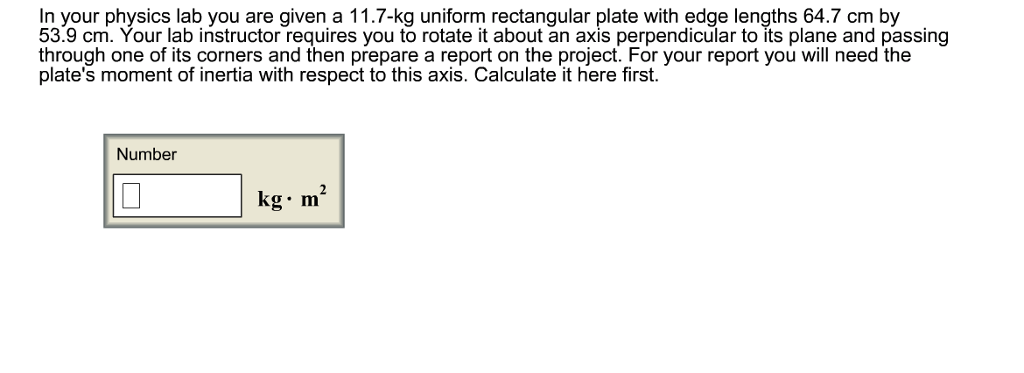In your physics lab you are given a 11.7-kg uniform rectangular plate with edge lengths 64.7 cm by 53.9 cm. Your lab instructor requires you to rotate it about an axis perpendicular to its plane and passing through one of its corners and then prepare a report on the project. For your report you will need the plate's moment of inertia with respect to this axis. Calculate it here first. Number kg m

• ### positive direction) Multiple Choice 15% each! 1. A solid uniform sphere of mass 1.85 kg and...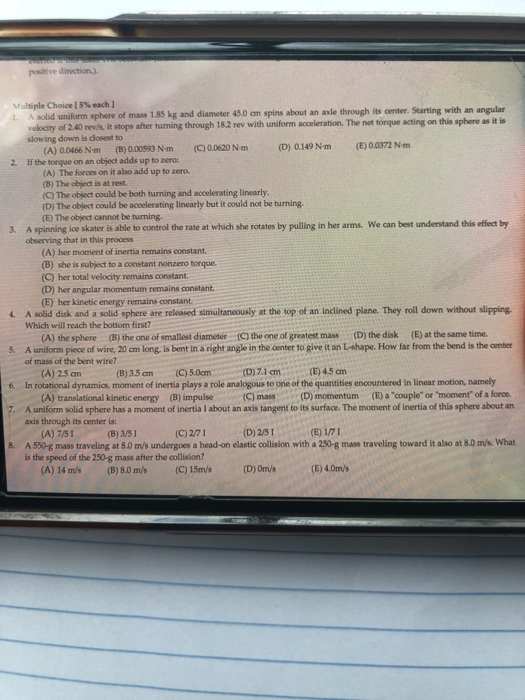positive direction) Multiple Choice 15% each! 1. A solid uniform sphere of mass 1.85 kg and diameter 45.0 cm spins about an axle through its center. Starting with an angular velocity of 2.40 revisit stops after turning through 18.2 rev with uniform acceleration. The net torque acting on this sphere as it is slowing down is dosest to (A) 0.0466 Nm (B) 0.00593 Nm (C) 0.0620 Nm (D) 0.149 Nm (E) 0.0372 Nm 2. If the torque on an object...

• ### Q21 (15 points): A uniform rod of mass m 1.5 kg and length d- 2.0 m...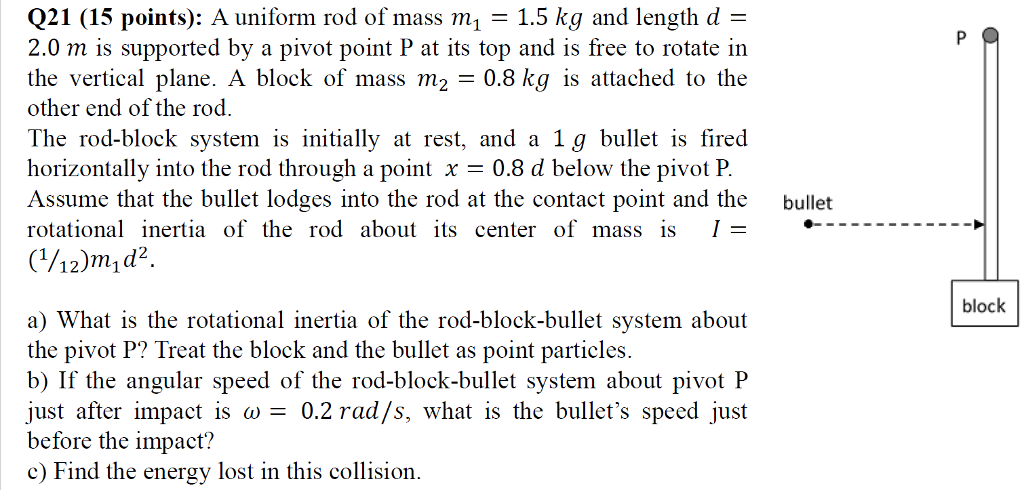Q21 (15 points): A uniform rod of mass m 1.5 kg and length d- 2.0 m is supported by a pivot point P at its top and is free to rotate iın the vertical plane. A block of mass m2 0.8 kg is attached to the other end of the rod. The rod-block system is initially at rest, and a 1g bullet is fired horizontally into the rod through a point x 08 d below the pivot P Assume that...

• ### O and block B has mass me 12.0Kg P A Rotation Dynamics 1. A uniform disk...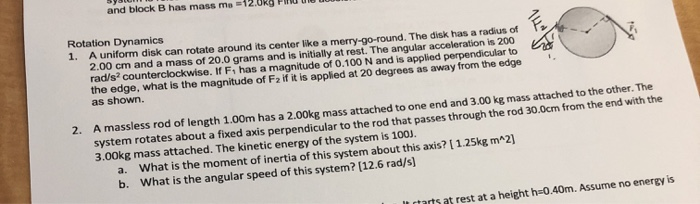O and block B has mass me 12.0Kg P A Rotation Dynamics 1. A uniform disk can rotate around its center like a merry-go-round. The disk has a radius of 2.00 cm and a mass of 20.0 grams and is initially at rest. The angular acceleration is 200 rad/s counterclockwise. If F has a magnitude of 0.100 N and is applied perpendicular to the edge, what is the magnitude of F2 if it is applied at 20 degrees as away...

• ### Block 1 has a mass of m1 = 450 g and Block 2 has a mass...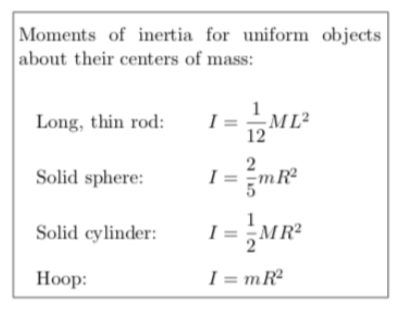Block 1 has a mass of m1 = 450 g and Block 2 has a mass of m2 = 500 g. The pulley, which is mounted on a horizontal axle with negligible friction through its center, has a radius of 5.00 cm. When released from rest, Block 2 accelerates downward at a rate of 0.425 m/s2 without the cord slipping on the pulley. What is the rotational inertia of the pulley? Moments of inertia for uniform objects about their centers...

Free Homework App